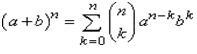# Revision Notes - Binomial Theorem Notes | Study Mock Test Series for JEE Main & Advanced 2022 - JEE

## JEE: Revision Notes - Binomial Theorem Notes | Study Mock Test Series for JEE Main & Advanced 2022 - JEE

The document Revision Notes - Binomial Theorem Notes | Study Mock Test Series for JEE Main & Advanced 2022 - JEE is a part of the JEE Course Mock Test Series for JEE Main & Advanced 2022.
All you need of JEE at this link: JEE
• If x, y ∈ R and n ∈ N, then the binomial theorem states that
(x+y)n = nC0xn + nC1 xn-1y+ nC2 xn-2 y+…… … .. + nCrxn-r y+ ….. + nCnyn
which can be written as ΣnCrxn-ryr. This is also called as the binomial theorem formula which is used for solving many problems.
• Some chief properties of binomial expansion of the term (x+y)n:
1. The number of terms in the expansion is (n+1) i.e. it is one more than the index.
2. The sum of indices of x and y is always n.
3. The binomial coefficients of the terms which are equidistant from the starting and the end are always equal. The simple reason behind this is
C(n, r) = C(n, n-r) which gives C(n, n) C(n, 1) = C(n, n-1) C(n, 2) = C(n, n-2).
• Such an expansion always follows a simple rule which is:
1. The subscript of C i.e. the lower suffix of C is always equal to the index of y.
2. Index of x = n – (lower suffix of C).
• The (r +1)th term in the expansion of expression (x+y)n is called the general term and is given by Tr+1 = nCrxn-ryr
• The term independent of x is obviously without x and is that value of r for which the exponent of x is zero.
• The middle term of the binomial coefficient depends on the value of n. There can be two different cases according to whether n is even or n is odd.
1. If n is even, then the total number of terms are odd and in that case there is a single middle term which is (n/2 +1)th and is given by nCn/2 an/2 xn/2.
2. On the other hand, if n is odd, the total number of terms is even and then there are two middle terms [(n+1)/2]th and [(n+3)/2]th which are equal to nC(n-1)/2 a(n+1)/2 x(n-1)/2 and nC(n+1)/2 a(n-1)/2 x(n+1)/2
• The binomial coefficient of the middle term is the greatest binomial coefficient of the expansion.
• Some of the standard binomial theorem formulas which should be memorized are listed below:
1. C+ C+ C2 + ….. + Cn= 2n
2. C+ C2 + C4 + ….. = C1 + C3 + C5 + ……….= 2n-1
3. C02 + C1+ C22 + ….. + Cn2 = 2nC= (2n!)/ n!n!
4. C0Cr + C1Cr+1 + C2Cr+2+ ….. + Cn-rCn=(2n!)/ (n+r)!(n-r)!
5. Another result that is applied in binomial theorem problems is nC+ nCr-1 = n+1Cr
6. We can also replace mC0 by m+1C0 because numerical value of both is same i.e. 1. Similarly we can replace mCm by m+1Cm+1.
7. Note that (2n!) = 2n. n! [1.3.5. … (2n-1)]
• In order to compute numerically greatest term in a binomial expansion of (1+x)n, find Tr+1 / Tr= (n – r + 1)x/r. Then put the absolute value of x and find the value of r which is consistent with the inequality Tr+1 / Tr> 1.
• If the index n is other than a positive integer such as a negative integer or fraction, then the number of terms in the expansion of (1+x)is infinite.
• The expansions in ascending powers of x are valid only if x is small. If x is large, i.e. |x| > 1 then it is convenient to expand in powers of 1/x which is then small.
• The binomial expansion for the nth degree polynomial is given by:• Following expansions should be remembered for |x| < 1:
1. (1+x)-1 = 1 – x + x– x3 + x4 - ….. ∞
2. (1-x)-1 = 1 + x + x2+ x+ x4+ ….. ∞
3. (1+x)-2 = 1 – 2x + 3x– 4x3 + 5x4 - ….. ∞
4. (1-x)-2 = 1 +2x + 3x2+4x+ 5x4+ ….. ∞
The document Revision Notes - Binomial Theorem Notes | Study Mock Test Series for JEE Main & Advanced 2022 - JEE is a part of the JEE Course Mock Test Series for JEE Main & Advanced 2022.
All you need of JEE at this link: JEEUse Code STAYHOME200 and get INR 200 additional OFF

## Mock Test Series for JEE Main & Advanced 2022

2 videos|258 docs|160 tests

Track your progress, build streaks, highlight & save important lessons and more!

,

,

,

,

,

,

,

,

,

,

,

,

,

,

,

,

,

,

,

,

,

;